Controlling The Real World With Computers
::. Control And Embedded Systems .::

Experiment 1 - Basic Switch Input Detection
 Home Order Let me know what you think

Previous: Putting It All Together - Controlling The Hardware With The Software
Next:
Experiment 2 - Expanding Switch Input Detection

The board is accessed through ports. Address Lines and Ports will tell you what ports are if you have forgotten.

Prototypes are often included for port input and output functions by including conio.h:
#include <conio.h>

The prototype for the function to read from a port is:
int inp(unsigned port);
This says that inp() returns an integer and has as its argument an unsigned integer with the port address. The returned integer is the contents of the port. Your compiler might use a different method for port input.

The prototype for the function to write to a port is:
int outp(unsigned port, int data);
This says that outp() returns an integer and has as its arguments an unsigned integer with the port address, plus the integer data that is to be sent out of the port. It returns the value sent. Your compiler might use a different method for port output.

Preparation of the Board for Experiment 1

NOTE: Please be sure to read the Warranty And Disclaimer before working with the hardware!

We will be looking at buffered data bits BD4, BD5 and BD6 from IC7: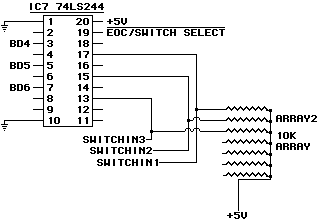Half of the 74LS244 is used. Pins 17, 15 and 13 are used as digital inputs, and are pulled up by ARRAY2 to permit their use with switches. Pins 17, 15 and 13 are routed through the buffers to pins 3, 5 and 7 respectively, provided they are activated (see the Boolean Logic section if you have forgotten about buffers). The output of the 74LS244 is placed on the data bus by taking pin 19 low: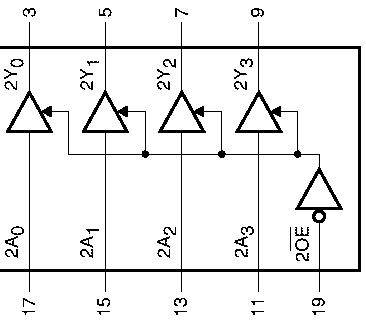The switch inputs are designated SWITCHIN1, SWITCHIN2 and SWITCHIN3 on the schematic and can be accessed on pins 5, 4 and 3 respectively of HEADER 1. Connecting one of the inputs to pin 1 or 2 of HEADER 1 will ground the input and pull it low to indicate that the switch has been turned on. Note that the dotted lines mean tha a connection is optional. One, none or all three of the swtiches may be connected to pin 1 or 2.:The following is part of the board layout showing the location of HEADER 1 at the top of the board: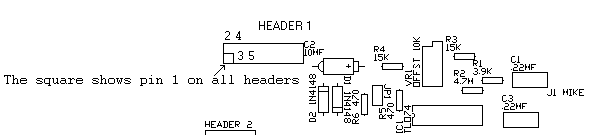The best way to connect to Header 1 is by means of a 16-pin 2-row female header connector with 8 pins on each row, .1" between pins and .1" between rows. Strip the ends of wires 1, 2, 3, 4 and 5. Look for the square pin on the back of the board. It is pin 1. Plug the header socket in so that the wire with the stripe is on the same end as the square pin. It's OK to use a header with more than 16 pins on header 1. Just let the end opposite the pin 1 end rest on C2.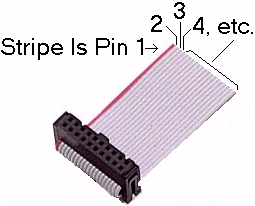If DIP address switches 1 and 2 are turned on and 3 is turned off on the board, its base port address will be 0x300, and the devices on the board will be in the following locations, as explained in the hardware section. You might find that there is a conflict with other devices on the computer. If so, change the switch settings to find an unused area. For example, 0x300 might be used by a sound card. If a different address is used, change all references to 0x300 to the new value. The hexadecimal notation used in the table is explained in Data lines, bits, nibbles, bytes, words, binary and HEX.

 Address Offset Device 0x300 ~ 0x307 0x00 Eight Channel Analog to Digital Converter 0x308 ~ 0x30F 0x08 Digital to Analog Converter 1 0x310 ~ 0x317 0x10 Digital to Analog Converter 2 0x318 ~ 0x31F 0x18 Analog to Digital Converter Ready Line And 3 Digital Inputs from IC7, a 74LS244 0x320 ~ 0x327 0x20 Programmable Peripheral Interface 0x328 ~ 0x32F 0x28 Spare Select Line 0x330 ~ 0x337 0x30 Spare Select Line 0x338 ~ 0x33F 0x38 Spare Select Line

Insert the board in one of the slots inside the computer. Install it so that the controls face the back of the computer. ALWAYS TURN OFF THE COMPUTER BEFORE INSTALLING A BOARD!!.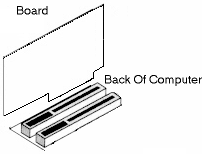The Software for Experiment 1

Let's design a function that returns a 1 if a particular switch is on, a 0 if it is off, and a -1 if an out-of-range switch number is asked for. Since we are asking if a switch has turned on, let's call it is_switch. An outline of the program might look like this:

```#include <stdio.h> // contains prototype for printf()
#include <conio.h> // contains prototype for inp()

int is_switch(int input); // prototype for is_switch

void main(void)
{
}

#define base 0x300 // change if 0x300 will not work
#define switch_port base + 0x18

int is_switch(int input)
{
}
```

Notice that the declarations of the base locations are below main(). This illustrates a concept called scope. Parts of the code above the declarations know nothing of the declared items. If they were variables this would isolate and protect them from modification by any functions but those below them. Their scope is at or below their declaration. As it is however, they are #defined by the #define compiler directive to be constants and can't be modified. Still, they are not known to functions above them. Notice that there is no colon at the end of the #define line.

The constant called base is declared as the base address. Since the base is at 0x300, with the DIP switch settings used and the switches are at 0x318, switch_port has been assigned to be the port address plus 0x18, or 0x318. Any of the DIP switch settings could have been used with a corresponding change to the declaration of base, and the one used for switches would still be base + 0x18. Change the base declaration and everything related to it changes with it.

The first order of business for is_switch() will be to check for inputs that are out of bounds. Switches other than 1, 2 or 3, will be rejected by returning a -1 to the calling function. The test will be accomplished with an if statement. if statements can ask many types of questions. Of concern here are queries comparing the values of integers. The queries, the symbols used for them and the C if statements are shown in the following table:

 Query in English Query Operator C if statement Is a equal to b? = = if(a = = b) Is a not equal to b? != if(a != b) Is a less than b? < if(a < b) Is a greater than b? > if(a > b) Is a less than or equal to b? <= if(a <= b) Is a greater than or equal to b? >= if(a >= b)

Be very careful with the first one. It is a common mistake for programmers to use a statement such as if(a = b). The single equal sign is an assignment operator, not a query operator.

The double equal (= =) is the query operator. It's the one that asks if two items are equal to each other. The error produced from using the single equal sign comes from the fact that the if(a = b) statement will always return true if a and b are the same variable types because a will simply get assigned the value of b. The if statement will ask if that happened, and the answer will be yes.

Notice that ! is the NOT query symbol.

We can use the above table to figure out what kind of question we want to ask in order to determine if the input value is out of range. Since we are dealing with switches 1, 2 and 3, we will not accept anything other than those numbers. We will return -1 if we get a number less than 1 or greater than 3.

One way to do it would be with two if statements:

```int is_switch(int input)
{
if(input < 1) // if the input is less than 1
return -1;  // return -1 showing an error

if(input > 3) // if the input is greater than 3
return -1;  // return -1 showing an error
}
```

Notice that there are no semicolons after the if statements. That's because the statements are not finished until action is taken as a result of the query. The if statements are indented to the right of the function's curley braces, and the return statements are indented to the right of the if statements for clarity. Indentations are a convenient way of showing one thing "belongs to" another. It's always a good idea to spread things out in order to make them as clear as possible. C doesn't care if you use white space for clarity, and humans appreciate it.

The above will work, but it would be nice if the whole less-than-1, greater-than-3 question could be on one line. What we really want to say is, "If the input is less than 1 OR greater than 3, then return a - 1." There are symbols that provide such capability. The double ampersand (&&) provides an AND, and the double bar (||) provides the OR. Please note however, that the use of a single symbol where a double symbol is required will create errors that the compiler will often not detect. The single versions are for Boolean operations (see the Boolean Logic section if you don't know what that means).

The function with the combined if statement now becomes:

```int is_switch(int input)
{
if(input < 1 || input > 3) // if the input is out of range,
return -1;               // return -1 showing an error
}
```

This says, "If the input is less than 1 OR the input is greater than 3, then return a -1."

It's a good idea to find the simplest logic available. The above rejects errors immediately and leaves the function. Rather than using a rejecting logic, an accepting logic could have been used:

```int is_switch(int input)
{
if(input >= 1 && input <= 3) // if the input is in range then do
{                            // everything inside the curley braces
// everything inside the curley braces belongs to the if statement
}

else return -1; // else return -1 showing an error
}
```

This says, "If the input is greater than or equal to 1 AND the input is less than or equal to 3, then do everything inside the curley braces, else return a -1 to show the input is out of range." This will work, but it's not as clean.

After checking to make sure the input is within bounds, we can get the switch input information by using the inp() function:
inp(switch_port);

This actually provides more information than required. Even though inp() returns an integer, all we need are the 3 bits in its least significant byte. The switches are related to the input byte we will use in the following manner:

```Bit       7    6    5    4    3    2    1    0
Switch         3    2    1
HEX Weight   0x40 0x20 0x10
```

We could use some if statements and some Boolean logic to test the individual bits to see if the appropriate one has been pulled low, indicating the switch has been turned on:

```int is_switch(int input)
{
if(input < 1 || input > 3) // if the input is out of range,
return -1;               // return -1 showing an error

if(input == 1)                    // do everything inside the following
{                                 // curley braces if the input is a 1
if(!(inp(switch_port) & 0x10)) // bit 4 is low
return 1;                     // so show switch 1 is on
}

else if(input == 2)               // else do everything inside the following
{                                 // curley braces if the input is a 2
if(!(inp(switch_port) & 0x20)) // bit 5 is low
return 1;                     // so show switch 2 is on
}

else if(input == 3)               // else do everything inside the following
{                                 // curley braces if the input is a 3
if(!(inp(switch_port) & 0x40)) // bit 6 is low
return 1;                     // so show switch 3 is on
}

return 0; // no switches on

}
```

Ok, so what does something like if( !(inp(switch_port) & 0x10) ) mean?! Let's build from what is known to what is needed.

The basic information about the switches is at the port location we have named switch_port, and can be obtained by calling inp():
inp(switch_port)
There is no need to set anything equal to inp(switch_port). The compiler knows that an internal temporary variable is to be used. It will save the return of inp(switch_port) in the temporary variable and then use it for other operations called for in the statement.

Let's say we want to know if switch 1 is turned on. The input argument would equal 1. Switch 1 information is at the bit 4 position.

Bit 4 is normally pulled high by a resistor. If switch 1 is turned on, it will ground the input and turn bit 4 off. These two states are illustrated in the following table. An x is placed in the bit positions we don't care about. Bit 4 is our only concern here.

 Data Bit D7 D6 D5 D4 D3 D2 D1 D0 Switch 1 Off x x x 1 x x x x Switch 1 On x x x 0 x x x x HEX Bit Weight 0x80 0x40 0x20 0x10 0x08 0x04 0x02 0x01

Now consider what happens if the value from switch_port is ANDed with 0x10, the weight for bit 4. In binary format, 0x10 is 00010000. Remember from the Boolean Logic section that ANDing two bytes simply involves ANDing their corresponding bits. Therefore, if bit 4 is high and the byte is ANDed with 0x10, the result will be 0x10. If bit 4 is low and the byte is ANDed with 0x10, the result will be 0. Thus, ANDing the value from switch_port with 0x10 gives us part of the information we need by masking off all other bits:
inp(switch_port) & 0x10

Since the goal is to know the condition of all of the above as a whole, wrap it in parenthesis:
(inp(switch_port) & 0x10)

What's needed is to know if it is true that the above is low. That logic flip is done by adding the NOT query symbol (!). The if statement is then applied to all of the above with the NOT symbol placed before the inside parentheses. There is an outside parentheses because the if statement requires it.

Now, if it is true that the information from switch_port ANDed with 0x10 is low, the if statement will return a true. That happens when switch 1 is turned on:
if( !( inp(switch_port) & 0x10 ) )

```Is it true that
| the following inside the parenthesis is low:
|  |   the data at     ANDed with 0x10 to mask off unwanted bits
|  |   switch_port     |          |
|  |       |           |          |
if(!( inp(switch_port) &         0x10) )
```

It would be nice if we could get away from all of the if statements about the input and simply switch among the three acceptable choices or reject errors. That's possible with the switch() statement:

```int is_switch(int input)
{
switch(input) // everything inside the curley braces
{             // belongs to the switch statement

case 1:                         // case where input == 1
if(!(inp(switch_port) & 0x10))  // bit 4 is low
return 1;                     // so return 1 showing switch 1 is on
return 0;                       // else show its off
break;                          // break away from this part
// of the switch statement

case 2:                         // case where input == 2
if(!(inp(switch_port) & 0x20))  // bit 5 is low
return 1;                     // so return 1 showing switch 2 is on
return 0;                       // else show its off
break;                          // break away from this part
// of the switch statement

case 3:                         // case where input == 3
if(!(inp(switch_port) & 0x40))  // bit 6 is low
return 1;                     // so return 1 showing switch 3 is on
return 0;                       // else show its off
break;                          // break away from this part
// of the switch statement

default:                        // the default condition is
// that the input is not 1, 2 or 3
return -1;                      // so return -1 to show out of bounds
break;                          // break away from this part
// of the switch statement

} // end of switch(input)

}
```

Notice the sequence in the first 3 cases. There is an if statement followed immediately by a return 1. Immediately after that, there is a return 0 and a break. You might see "else return 0" in some software even though the "else" part is not necessary. Problem is, it often is necessary, so people use it as a matter of form. Also, if you study the statements carefully, you will find that the "break;" part is not needed. It is also used for good form. It tells the reader the case statement is over. Besides, it's absolutely necessary in most cases.

The above will work, but it's actually a little messier than multiple if() / else if statements. It's useful mainly to show how switch() works. Let's take another look at what we can get with inp() compared to what we need. As already noted, the switches are related to the input byte in the following manner:

```Bit       7    6    5    4    3    2    1    0
Switch         3    2    1
HEX Weight   0x40 0x20 0x10
```

One of the things that's needed for the test is to AND the correct weight with the byte from switch_port. It would be nice to be able to simply shift a 1 into the correct bit position. Remember from Data lines, bits, nibbles, bytes, words, binary and HEX that bit 0 has a weight of 1. If bit 0 is set to 1, shifting it left 4 will provide 0x10, shifting it left 5 makes it 0x20 and shifting it left 6 will give us 0x40. Thus, the correct weight value can be obtained by shifting a 1 to the left by the input value plus 3. The C left shift operator (<<) will do just that. Thus, the input will be ANDed with the correct weight by the following operation:
inp(switch_port) & (1 << (input + 3))

That's ok, but it would be even better if we could shift the bit we need down to the 0th bit position so we are always dealing with a 1. That's also possible with the right shift operator (>>). With it, the following can be done:
inp(switch_port) >> (input + 3)
This will cause the results of inp(switch_port) to be shifted to the right 4, 5 or 6 bits depending on whether the input is for switch 1, 2 or 3, thus placing the desired bit into bit position 0.

Now all we have to do is AND the result with 1 rather than worrying about a specific weight value:
(inp(switch_port) >> (input + 3)) & 1
ANDing with 1 will be correct regardless of the particular switch status being requested. It will mask off everything but what is needed to make a determination.

The only problem remaining is that the above will return the wrong polarity. If the switch is on, bit 0 will be low. ANDing with 1 will produce a 0. If the switch is off, bit 0 will be high. ANDing with 1 will produce 1. The XOR operation , discussed in the Boolean Logic section, can be used to produce the desired return value. If the AND operation produces a 1, XORing with 1 will produce a 0. If the AND operation produces a 0, XORing with 1 will produce a 1:
return ((inp(switch_port) >> (input + 3)) & 1) ^ 1;

To outline what the above says:

1. Get the data from switch_port with inp(switch_port).

2. Shift the results of the above to the right (input + 3) bits. This will place the desired bit in the bit 0 position.

3. AND the results of the above with 1 to mask off everything but bit 0.

4. XOR the results of the above with 1 to reverse the polarity.

5. Return the resulting 1 or 0.

``` return     the data from    shifted right     ANDed     and all of
|         switch_port     input + 3 bits    with 1    this XORed
|             |                 |             |       with 1 to change polarity
|             |                 |             |         |
return ((inp(switch_port)   >> (input + 3))     & 1)      ^ 1;
```

The next task is to test is_switch() using main() to send data that includes both legal and illegal inputs. A while() loop will be used to continuously call is_switch(). A while() loop does what is sounds like it would do -- it keeps going as long as some condition is met. For example, a while() loop could be used to simulate the for() loop discussed earlier:

```x = 0; // first set x to start at 0

while(x < 11) // do everything in the while() loop's curley
{             // braces as long as x is less than 11

printf("x = %d\n",x); // print x
x++;                  // bump x up one

} // end of while(x < 11)
```

To test is_switch() we will use a for() loop nested inside a while() loop:

```while(1) // since 1 is always 1, this while() loop will run forever
{
if(kbhit()) // "keyboard hit" -- a compiler function that sees if a key has been
break;    // pressed.  We will break out of the while() loop if a key is pressed.

for(x=0; x<5; x++) // purposely use numbers less than 1 and greater than 3
{
y = is_switch(x);
// perform other operations here
}

} // end while(1)
```

The program used to test the final version of is_switch() for this section is below:

 ``` // experi1.c #include // contains prototype for printf() and puts() #include // contains prototypes for inp() and kbhit() int is_switch(int input); // prototype for is_switch void main(void) { int x,y; while(1) // since 1 is always 1, this while() loop will { // run until a break command is called if(kbhit()) // "keyboard hit" -- a compiler function that sees if a key has been break; // pressed. We will break out of the while() loop if a key is pressed. for(x=0; x<5; x++) // purposely use numbers less { // than 1 and greater than 3 y = is_switch(x); printf("x = %d y = %2d | ",x,y); // don't print a new line yet } puts(""); // puts("") will put a new line on the screen // after all 5 lines have been printed } // end while(1) } #define base 0x300 // change if a conflict is found #define switch_port base + 0x18 /* ============================================================================= is_switch() is_switch() returns -1 if the input is less than 1 or greater than 3. It returns 1 if the switch number designated by input is on, else it returns 0 using the following procedure: 1. Get the data from switch_port with inp(switch_port). 2. Shift the results of the above to the right (input + 3) bits. This will place the desired bit in the bit 0 position. 3. AND the results of the above with 1 to mask off everything but bit 0. 4. XOR the results of the above with 1 to reverse the polarity. 5. Return the resulting 1 or 0. ============================================================================= */ int is_switch(int input) { if(input < 1 || input > 3) // if the input is less than 1 or greater return -1; // than 3, then return -1 showing an error return ((inp(switch_port) >> (input + 3)) & 1) ^ 1; } // end is_switch() // end experi1.c ```

To compile experiment 1 using the MIX PowerC compiler, type in the following then press the enter key:
\powerc\pc /e /n /ml experi1

This translates to PowerC Compile, linking to make an executable (/e), allowing nested comments (comments within comments /n) and the large memory model (/ml) for unlimited code and data space.